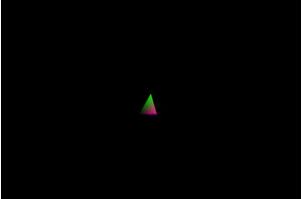modern opengl tutorial

end

# 第十四课 相机控制（一）

## 代码

``````(Camera.h)
class Camera
{
public:
Camera();
Camera(const Vector3f& Pos, const Vector3f& Target, const Vector3f& Up);
bool OnKeyboard(int Key);
const Vector3f& GetPos() const
const Vector3f& GetTarget() const
const Vector3f& GetUp() const
private:
Vector3f m_pos;
Vector3f m_target;
Vector3f m_up;
};``````

``````(Camera.cpp:42)
bool Camera::OnKeyboard(int Key)
{
bool Ret = false;
switch (Key) {
case GLUT_KEY_UP:
{
m_pos += (m_target * StepSize);
Ret = true;
}
break;
case GLUT_KEY_DOWN:
{
m_pos -= (m_target * StepSize);
Ret = true;
}
break;
case GLUT_KEY_LEFT:
{
Vector3f Left = m_target.Cross(m_up);
Left.Normalize();
Left *= StepSize;
m_pos += Left;
Ret = true;
}
break;
case GLUT_KEY_RIGHT:
{
Vector3f Right = m_up.Cross(m_target);
Right.Normalize();
Right *= StepSize;
m_pos += Right;
Ret = true;
}
break;
}
return Ret;
}``````

``````(tutorial14.cpp:73)
static void SpecialKeyboardCB(int Key, int x, int y)
{
GameCamera.OnKeyboard(Key);
}
static void InitializeGlutCallbacks()
{
glutDisplayFunc(RenderSceneCB);
glutIdleFunc(RenderSceneCB);
glutSpecialFunc(SpecialKeyboardCB);
}``````

``````(tutorial14.cpp:55)
p.SetCamera(GameCamera.GetPos(), GameCamera.GetTarget(), GameCamera.GetUp());``````

## 操作结果